# Wigner-Weyl transform

(diff) ← Older revision | Latest revision (diff) | Newer revision → (diff)

Weyl–Wigner transform

Let,, be a ray in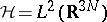. Then, for each, the Wigner transform ofiswhereis Planck's constant. The quantityis called the Wigner function. It was introduced by E.P. Wigner in 1932, [a1], who interpreted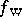as a quasi-probability density in the phase spaceand showed that it obeyed a kinetic pseudo-differential equation (the Wigner equation) of the form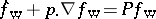, whereis a pseudo-differential operator with symbol defined by the potential energy of the system. Wigner went on to discuss howmight be used to calculate quantities of physical interest. In particular, the density is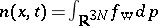. Since, in general, the potential energy depends on the density, the Wigner equation is non-linear.

Generalizing to a mixed state, described not by a wave function but by a von Neumann density matrix [a2]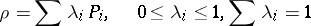(is the projection onto the vector):Generalizing further, letbe a (bounded) operator on. Let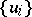be a basis for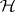and write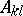for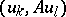, where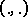is the inner product in. Then the Wigner transform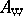of the operatoris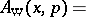In particular, ifis a trace-class operator onandis bounded as above,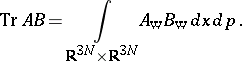The Wigner transform of an operator is related to the Weyl transform [a3] of a phase-space function, introduced by H. Weyl in 1950 in an attempt to relate classical and quantum mechanics. Indeed, letbe an appropriate function in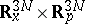(see [a4] for a definition of "appropriate" ). Then the Weyl transform of,, is defined in terms of the Fourier transformofas [a5]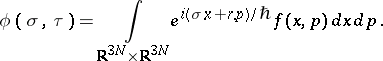Here,is the operatorandis the multiplication operator on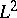defined by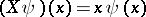and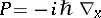. These are the usual position and momentum operators of quantum mechanics [a2]. The Weyl and Wigner transforms are mutual inverses:and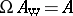[a5].

Serious mathematical interest in the Wigner transform revived in 1985, when H. Neunzert published [a6]. Since then, most mathematical attention has been paid to existence-uniqueness theory for the Wigner equation in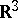and, more recently, in a closed proper subset of,. While the situation inis pretty well understood, [a7], [a8] the more practical latter situation is still under study (1998), the main problem being the question of appropriate boundary conditions [a9].

How to Cite This Entry:
Wigner-Weyl transform. Encyclopedia of Mathematics. URL: http://encyclopediaofmath.org/index.php?title=Wigner-Weyl_transform&oldid=11631
This article was adapted from an original article by P.F. Zweifel (originator), which appeared in Encyclopedia of Mathematics - ISBN 1402006098. See original article